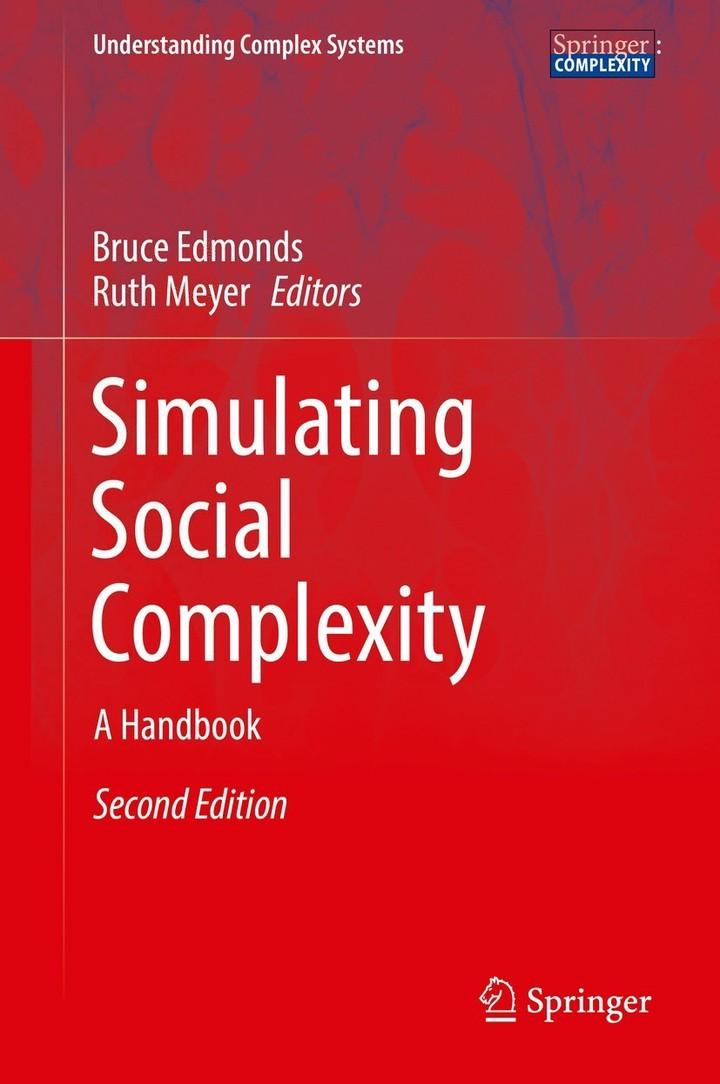# How many times should one run a computational simulation?### Abstract

This chapter is an attempt to answer the question “how many runs of a computational simulation should one do,” and it gives an answer by means of statistical analysis. After defining the nature of the problem and which types of simulation are mostly affected by it, the chapter introduces statistical power analysis as a way to determine the appropriate number of runs. Two examples are then produced using results from an agent-based model. The reader is then guided through the application of this statistical technique and exposed to its limits and potentials.

Publication
Simulating Social Complexity - A Handbook, 2nd edition, edited by B. Edmonds and R. Meyer, Springer-Verlag Berlin Heidelberg, pp. 229-251##### Raffaello Seri
###### Professor of Econometrics

My research interests include statistics, numerical analysis, operations research, psychology, economics and management.